Home > CCA2 > Chapter 9 > Lesson 9.1.1 > Problem9-13

9-13.
1. The Richter scale assigns a magnitude number to quantify the energy released by an earthquake. The magnitude of an earthquake was defined by Charles Richter to be M = log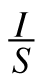where I is the intensity of the earthquake (measured by the amplitude of a seismograph reading taken 100 km from the epicenter of the earthquake) and S is the intensity of a “standard earthquake” (whose amplitude is 1 micron = 10–4 cm). For example, an earthquake measuring 3.6 on the Richter scale is 100.2 or approximately 1.6 times as intense as an earthquake measuring 3.4 on the Richter scale. Homework Help ✎

1. How many times stronger is an earthquake that measures 6.5 on the Richter scale than an earthquake that measures 5.5?

2. How many times stronger is an earthquake that measures 5.1 than an earthquake that measures 4.3? Give your answer both as a power of 10 and as a decimal. Make sure your answer has the same level of precision as the Richter measurements.

3. What is the magnitude of an earthquake that is half as strong as an earthquake measuring 6.2 on the Richter scale?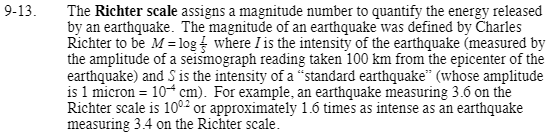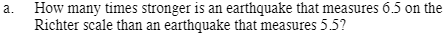10 times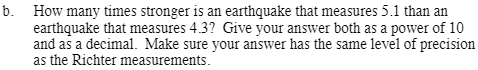100.8 = 6.3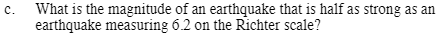Calculate the energy released by a Richter measurement of 6.2. Then divide in half.

$10^?=10^\frac{6.2}{2}$

You may want to use logs or guess and check to find the answer.